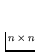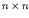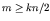# Computer Science 547 - Discrete Mathematics Prof. Steven Skiena Fall 1992

Homework 5
Due Tuesday, April 27, 1999

Each of the problems should be solved on a separate sheet of paper to facilitate grading. Limit the solution of each problem to one sheet of paper. Staple all these sheets together! Please don't wait until the last minute to look at the problems.

1.
The complement G' of a graph has the same vertices of G but an edge if and only if G does not. A graph G is self-complementary if G is isomorphic to its complement. Show that if G is self-complementary, then the number of vertices is congruent to 0 or 1 mod 4.

2.
Anchessboard defines a rooks-tour graph Rn, withvertices corresponding to squares and two vertices are connected by an edge iff the associated squares can be the starting and ending points of a single rook move. Note that this is NOT angrid.

a) For what values of n is Rn Eulerian?

b) For what values of n is Rn Hamiltonian?

c) For what values of n is Rn planar?

d) For what values of n does Rn have a perfect matching?

e) What is the chromatic number of Rn?

f) What is the vertex connectivity of Rn?

3.
Let a graph G have at least one edge. The set of vertices of the line graph of G, denoted L(G), consists of the edges of G, with two nodes of L(G) adjacent whenever the corresponding edges of G are. For example, the line graph of a simple cycle on n vertices is isomorphic to itself, while the line graph of a simple path on n vertices is a simple path on n-1 vertices.

(a) Prove that if G is Eulerian, then L(G) is Eulerian and Hamiltonian.

(b) Prove that if G is Hamiltonian, then L(G) is Hamiltonian.

4.
Show that if a graph G with n vertices and m edges is k-edge-connected, then.

5.
An (f,d)-regular polyhedron graph is a plane graph with each vertex of degree d such that each face as f sides. Use Euler's formula (v - e + r = 2) to show that there are exactly five such graphs, the largest of which is the dodecahedron, the (5,3)-regular polyhedron graph.

6.
Prove that every planar graph is six-colorable, without resorting to the proof given in class that every planar graph is five-colorable. For extra credit, prove that every planar graph is four-colorable (JOKE!!).

7.
Prove that any tree has at most one perfect matching.